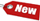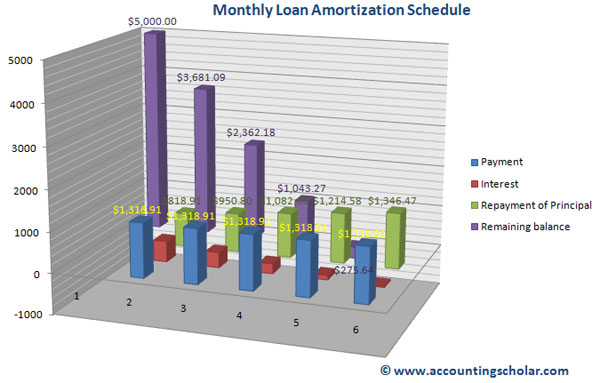Browse Accounting Lessons HereAccounting Terms & DefinitionsAccounting for Merchandising ActivitiesDebits and Credits (Double Entry Accounting)Business Valuation FormulasTime Value of Money & Present/Future ValuesComplex Debt & Equity InstrumentsCommon Stock & Shareholder's EquityAccounting & Finance RatiosValuing Common StockCorporate Income TaxesLower of Cost or Market (LCM) & Inventory ValuationChart of Accounts & BookkeepingBonds Payable & Long Term LiabilitiesCapital AssetsGAAP, Accrual & Cash Accounting, Information Commodity, Internal Controls & MaterialityWhat category of browser are you on this website? Accounting student (homework help) Finance professor (university research) Accounting manager (at work) Other Explore Careers in Accounting and FinanceVisit our section on Careers in Accounting & Finance to explore vast opportunities in this industry.

Amortized Loan - How to Calculate Loan Amortization using Present Value Interest Factor

An amortized loan is one that is paid off in equal periodic instalments or payments and includes varying portions of principal & interest during its term. Examples of amortizable loans include auto loans, mortgages, business loans & others. How do you compute the periodic payments on an amortized loan?

The formula is:

 Amount of loan = A = (P / PVIFA)

Where:

 P = Principal loan amount PVIFA = Present value interest factor

How to calculate the amount of loan:

1) Divide the principal loan amount (A) b PVIFA, which is a factor shown in the Present Value Interest

Factor of Annuity of \$1 table, and use this formula

 Amount of loan = A = (P / PVIFA)

Example

Consider a firm borrows \$5,000 to be repaid in five equal instalments at the end of each of the next five years. The discount rate on this loan payable is 10%. What is the amount of each instalment payment?

 P = \$5,000 PVIFA (10%, 5 years) = 3.791 (using the present value interest factor of \$1 annuity table) Amount of loan = A = (P / PVIFA) Amount of loan = A = \$5,000 / 3.791 Amount of loan = A = \$1318.91

Once the monthly loan instalment is computed, a loan amortization schedule can be created and each loan payment consists partly of interest & principal. The interest portion of the payment is usually the largest during the first few periods because the loan amount is highest at that point in time. However, as instalments are made and the loan balance decreases, the interest payments become lower as the interest rate is updated with the ending balance of the loan amount. Confused? We derive an example below:

 Year Payment (B) Interest (C) Repayment of Principal (D) = (B) – (C) ** Ending Balance (E) 0 \$5,000.00 1 \$1,318.91 \$500.00 * \$818.91 \$3,681.09 2 \$1,318.91 \$368.11 \$950.80 \$2,362.18 3 \$1,318.91 \$236.22 \$1,082.69 \$1,043.27 4 \$1,318.91 \$104.33 \$1,214.58 -\$275.64 5 \$1,318.91 -\$27.56 \$1,346.47

* Note the interest in Year 1 is calculated by multiplying the ending balance, \$5000 by 10% which totals \$500. In year 2, interest is calculated by multiplying the ending balance \$3,681.09 by 10% which is \$368.11.

** Repayment of Principal (D) = (B) – (C) ----We know that the total monthly payment will be \$1,318.91, thus the repayment of principal amount is Payment – Interest.Mortgage lenders who work for banks & financing companies use this amortization formulas and methods to determine the periodic mortgage payments & break them down in to principal & interest reductions. This information is also used by consumers who need a breakdown of their monthly payments, and how much of that goes in to interest & how much balance goes towards their principal.